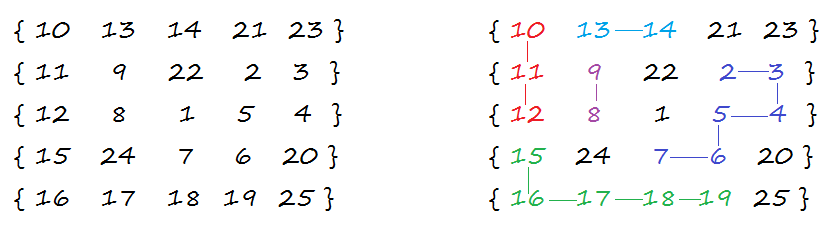# Find longest sequence formed by adjacent numbers in the matrix

Given a N x N matrix where each cell has distinct value in the 1 to N * N. Find the longest sequence formed by adjacent numbers in the matrix such that for each number, the number on the adjacent neighbor is +1 its value.

For example, if we are at location (x, y) in the matrix, we can move to (x, y + 1), (x, y – 1), (x + 1, y) or (x – 1, y) if the value at destination cell is one more than the value at source cell. The longest sequence formed by adjacent numbers in below matrix is 2 – 3 – 4 – 5 – 6 – 7The idea is to use recursion. The problem has an optimal substructure. That means the problem can be broken down into smaller, simple “subproblems”, which can further be divided into yet simpler, smaller subproblems until the solution becomes trivial. The problem can be recursively defined as –

longest path starting from cell (i, j) =

M[i][j] + | longest path starting from cell (i-1, j) (if M[i][j]+1 = M[i-1][j])
| longest path starting from cell (i, j-1) (if M[i][j]+1 = M[i][j-1])
| longest path starting from cell (i, j+1) (if M[i][j]+1 = M[i][j+1])
| longest path starting from cell (i+1, j) (if M[i][j]+1 = M[i+1][j])

## C++

Output:

2 – 3 – 4 – 5 – 6 – 7

## Java

Output:

2 – 3 – 4 – 5 – 6 – 7

The implementation that involves only finding the length of longest sequence can be seen here.

The time complexity of above solution is exponential as we’re doing a lot of redundant work. As we are calculating the longest path starting from each cell (i, j) of the matrix. So,

longest path starting from 2 is (2 – 3 – 4 – 5 – 6 – 7)
longest path starting from 3 is (3 – 4 – 5 – 6 – 7)
longest path starting from 5 is (5 – 6 – 7)
longest path starting from 4 is (4 – 5 – 6 – 7)
longest path starting from 6 is (6 – 7)

longest path starting from 2 is (2 – 3 – 4 – 5 – 6 – 7)
longest path starting from 3 is (3 – 4 – 5 – 6 – 7)
longest path starting from 5 is (5 – 6 – 7)
longest path starting from 4 is (4 – 5 – 6 – 7)
longest path starting from 6 is (6 – 7)

longest path starting from 2 is (2 – 3 – 4 – 5 – 6 – 7)
longest path starting from 3 is (3 – 4 – 5 – 6 – 7)
longest path starting from 5 is (5 – 6 – 7)
longest path starting from 4 is (4 – 5 – 6 – 7)
longest path starting from 6 is (6 – 7)

longest path starting from 2 is (2 – 3 – 4 – 5 – 6 – 7)
longest path starting from 3 is (3 – 4 – 5 – 6 – 7)
longest path starting from 5 is (5 – 6 – 7)
longest path starting from 4 is (4 – 5 – 6 – 7)
longest path starting from 6 is (6 – 7)

As we can see, the same sub-problems are getting computed again and again. As the recursion grows deeper, more and more of this type of unnecessary repetition occurs. We know that problems having optimal substructure and overlapping subproblems can be solved by dynamic programming, in which subproblem solutions are memoized rather than computed again and again. Now each time we compute the longest path starting from cell (i, j), we save it. If we are ever asked to compute it again, we simply give the saved answer, and do not recompute it.

## C++

Output:

2 – 3 – 4 – 5 – 6 – 7

## Java

Output:

2 – 3 – 4 – 5 – 6 – 7

The time complexity of above solution is O(N2).

Exercise: Extend the solution to consider diagonal moves as well.(1 votes, average: 5.00 out of 5)Loading...